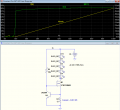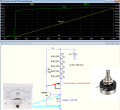# How does this constently maintain voltage on LEDs

#### ranatungawk

Joined Oct 30, 2008
198

#### Attachments

• 955.5 KB Views: 16

#### ericgibbs

Joined Jan 29, 2010
18,253
Hi rana,
The Constant Current set by R5

Constant current means just that, whether it's one or 5 LED's in series.

E

•drjohsmith and ranatungawk

#### Alec_t

Joined Sep 17, 2013
14,009
Constant current and constant voltage are two different things. The voltage across a LED is not constant, but depends somewhat on the current through it. The RM9003 contains transistors which adjust the voltage they supply to the LEDs so as to ensure the current is constant.

•ranatungawk

#### ranatungawk

Joined Oct 30, 2008
198
Hi rana,
The Constant Current set by R5

Constant current means just that, whether it's one or 5 LED's in series.

E
correct and understood. but how does this mosfet maintain constant current on the LED while the input is being changed from 6v to 600v?

#### ericgibbs

Joined Jan 29, 2010
18,253
hi,
When you have a LED load voltageat say 12V in total, there has to be an overhead voltage on the input to the IC, say 15V, this overhead voltage is called the compliance voltage, which means the Supply voltage must exceed the total voltage drop of the LED load by at least that voltage.

E

Amount of selection：
Because the IC bear the residual voltage in the circuit, so in the design of the voltage of
the LED string closer to AC rectifier after the voltage, so that the whole circuit running
efficiency to achieve the best. Proposed chip power consumption is less than 1.5W.
The AC input voltage (V rms), single LED grain to drop (V), forward current (m A). Then
design the total number of LED grains：
N =
（쳌䁠× 1.414 − ）

Last edited:
•ranatungawk

#### ranatungawk

Joined Oct 30, 2008
198
Constant current and constant voltage are two different things. The voltage across a LED is not constant, but depends somewhat on the current through it. The RM9003 contains transistors which adjust the voltage they supply to the LEDs so as to ensure the current is constant.
this is what i ask! does RM9003 get a reference from LED side to maintain current

#### ronsimpson

Joined Oct 7, 2019
2,797
This simple circuit is very close to how the IC works. The "+20V Supply" = " 3V - 300V or 6 to 600 ". R1=RS,
R2 turns on the MOSFET.
BC108C transistor turns on when the LED current produces 0.6V across R1 or RS. With the small transistor on the Gate voltage is pulled low and turns off the MOSFET. This makes a constant current circuit.The data sheet said it works to 6V but that depends on what LEDs you are using. With 10V of LEDs the IC needs (6V+10V=16V) minimum to work.
also:
Because it is often used without capacitors, the IC cannot make current during the zero crossing time of the power line.

•ranatungawk

#### MisterBill2

Joined Jan 23, 2018
16,621
this is what i ask! does RM9003 get a reference from LED side to maintain current
The reference that the current measurement is compared to is set by R5, as shown. The measurement of current is internal to the IC itself. So it is a problem resistant design, in that the sensing circuit is internal to the IC. But it is limited in the maximum power it can handle, which means that there is a maximum voltage limit for the voltage supplied to the string .

#### crutschow

Joined Mar 14, 2008
33,365
This simple circuit is very close to how the IC works
For that circuit to work it needs an N-MOSFET, with the drain connected to the LEDs.

Sim of example circuit below:
Note how the LED current (green trace) stays relatively constant once the supply voltage (horizontal axis) reaches the combined LED voltage of about 10V.
Q4 adjusts the MOSFET gate-source voltage to keep the current constant, meaning the drain voltage (yellow trace) changes to keep the current constant through the LEDs (and thus also the voltage across them).Last edited:
•ranatungawk and ronsimpson

#### ranatungawk

Joined Oct 30, 2008
198
hi,
When you have a LED load voltageat say 12V in total, there has to be an overhead voltage on the input to the IC, say 15V, this overhead voltage is called the compliance voltage, which means the Supply voltage must exceed the total voltage drop of the LED load by at least that voltage.

E

Amount of selection：
Because the IC bear the residual voltage in the circuit, so in the design of the voltage of
the LED string closer to AC rectifier after the voltage, so that the whole circuit running
efficiency to achieve the best. Proposed chip power consumption is less than 1.5W.
The AC input voltage (V rms), single LED grain to drop (V), forward current (m A). Then
design the total number of LED grains：
N =
（쳌䁠× 1.414 − ）
Can this be explain in "V=IR"

further, why it doesn't need to change the value of RS as the changes of the input voltage ? (6-600v)

Last edited:

#### ronsimpson

Joined Oct 7, 2019
2,797
Can this be explain in "V=IR"
yes
why it doesn't need to change the value of RS as the changes of the input voltage ? (6-600v)
RS is how you measure current.
The IC tries to hold 0.6V across RS. If the voltage is less than 0.6 it turns on (harder) internal MOSFET. If the voltage is above 0.6V it reduces the current in the MOSFET. You know V=0.6, current = 13 ohms, current = 50mA. The supply voltage is not in the formula. (assuming the supply is within range)

#### crutschow

Joined Mar 14, 2008
33,365
why it doesn't need to change the value of RS as the changes of the input voltage ? (6-600v)
In my simulation R4 performs the same function as RS.
The negative feedback from Q4 is such as to maintain a constant voltage across R4 (V = Id / R4) of about 0..65V, which maintains the constant-current, independent of Vs.

•ranatungawk

#### ranatungawk

Joined Oct 30, 2008
198
yes

RS is how you measure current.
The IC tries to hold 0.6V across RS. If the voltage is less than 0.6 it turns on (harder) internal MOSFET. If the voltage is above 0.6V it reduces the current in the MOSFET. You know V=0.6, current = 13 ohms, current = 50mA. The supply voltage is not in the formula. (assuming the supply is within range)
Dear ronsimpson, I feel answer is in your reply. but it's not clear to me. can you please explain it more ? what is the relationship between "0.6V across RS" and numbers of LED added ?

other than RM9003 , what are the equivalents?

#### crutschow

Joined Mar 14, 2008
33,365
what is the relationship between "0.6V across RS" and numbers of LED added ?
None.
RS establishes the current independent of the number of LEDs by maintaining 0.6V across RS, which is carrying the LED current.
That's why it's called a constant-current circuit.

•ranatungawk

#### BobTPH

Joined Jun 5, 2013
8,120
Let’s try another approach to explaining this since the previous attempts do not seem to satisfy you.

In the region of operation called the saturation region of a MOSFET, it is passes a specific current that depends in the gate voltage, no matter what the voltage between the source and drain are.

This property is what allows its use as a constant current sink. Set a particular gate voltage, and you will get nearly the same current whether the voltage drop from the drain to the sieve is. 3V or 300V.

Now, we don’t actually know what this gate voltage is for any given current. It will actually vary from one to another of the same model MOSFET. This is why we use negative feedback to set the current. A sense resistor is placed in the current path and it develops a voltage proportional to the current. That voltage is used in a circuit to adjust the gate voltage such that the voltage across the resistor is some constant. You can see all that in the simple circuit posted here. The 0.6V is the base to emitter voltage of a BJT. When it is higher the BJT conducts more current and pulls down the gate voltage. When the sense resistor voltage is lower than 0.6V the BJT conducts less current and the gate voltage rises.

Last edited:

#### crutschow

Joined Mar 14, 2008
33,365
other than RM9003 , what are the equivalents?
Some examples here.

History was made by those who broke the rules..
True.
But some of that history is less than admirable.#### AnalogKid

Joined Aug 1, 2013
10,792
History was made by those who broke the rules..
And many of those, died.

•ranatungawk

#### ronsimpson

Joined Oct 7, 2019
2,797
I am reposting this picture from crutschow but I removed the MOSFET and replaced it with a Pot (variable resistor).
It appears that the LEDs require about 2V/LED to get them to glow.
The Pot adds some amount of resistance to control the amount of current through the LEDs.
Power flows from the supply (Vs) through the LEDs, through the Pot, through R4 or Rs to ground. (R4 is like a current meter)
Q4 looks for the right amount of current flow. (0.6V across a resistor) Q4 "turns" the pot to get the right amount of resistance to get the right amount of current.But some of that history is less than admirable.